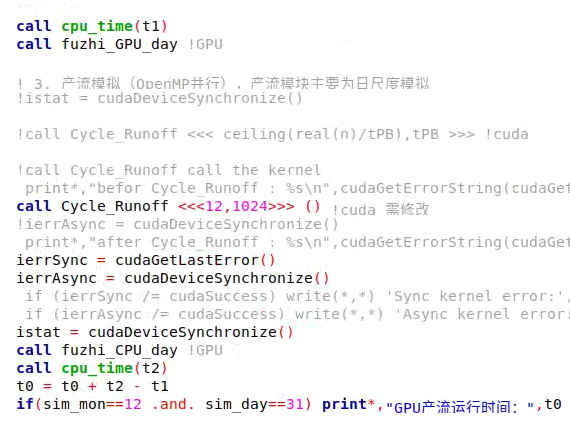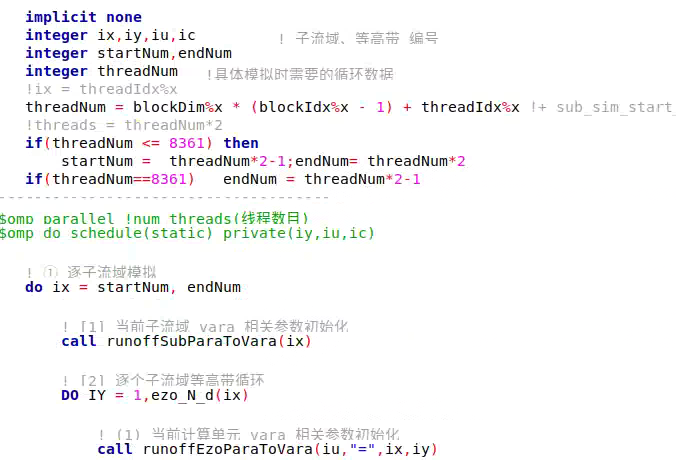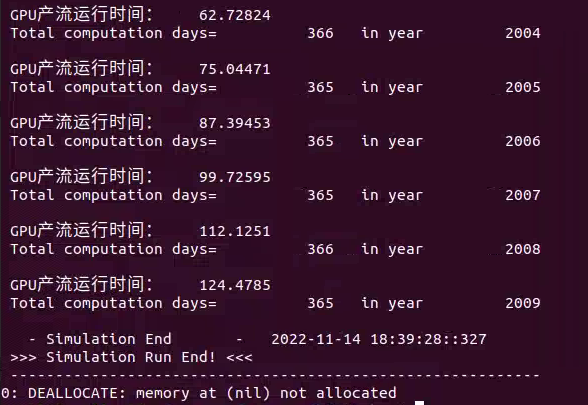# Nvfortran error

Hi Mat,
I encountered an nvfortran error：nvfortran-f-0155-compiler failed to translate accelerator region （see-minfo message）：device compiler exited with error status code,can you help me?

Hello, I have solved this problem because I wrote a piece of code repeatedly, but now I have some problems. I wonder if you can provide some resources? Can nvfortran use the trim function? Does nvfortran support len_ The function of trim and len to calculate string length?

In host code or device code? Yes for host code. I’ll have to check for device code. In general, our device code support for Fortran character data is not complete.

My current code includes the parallel code on the CPU and GPU. In my code, the device code does not recognize trim. It is always recognized as trima, but my host code does not recognize trim. Normally, trim, len, and len_ Trim is an internal function of fortran, shouldn’t it support nvfortran?

My window runs like this：

This fragment of my code is like this：

Under normal circumstances, the result of running my window should be that there is no blank line between 3, 4 and 5. I don’t know what is going on. Can you help me?

I’d really need a test I can compile and run myself. I do not see how wep_set_fold is set. And, line 343 is overwriting what is set in line 342.

Thank you bleblack, I have solved it. When I use nvfortran to compile and run my program, in the kernel function configuration, when the grid value is set to 2, 4, 6, 8, and 12, the running time is almost the same. When the number of threads is changed to 1024, the running time does not change much. What is the matter? The code defined by my kernel function and the running results are as follows:Hi 1799336883,

Without a reproducing example, we can’t give a definite answer. Though some possible reasons are:

• The calls to fuzhi_GPU_day and fuzhi_CPU_day dominate the time so the time spent in Cycle_Runoff doesn’t matter
• There not enough work in Cycle_Runoff so increasing the grid or block dimensions doesn’t matter.
• There’s some issue with Cycle_Runoff

Have you profile your code with Nsight-Systems and Nsight-Compute to get a more accurate view of the performance?

-Mat

Hello,Mat, I have solved this problem, and now I have a new problem,I want to ask you some questions. Can nvfortran compile openmp statements? I want to use cuda fortran and openmp to rewrite my fortran serial code under linux.

Can nvfortran compile openmp statements?
Yes.

I want to use cuda fortran and openmp to rewrite my fortran serial code under linux.

That’s fine, though if you’re wanting to use OpenMP to support multi-GPU programming, I prefer using MPI instead. With OpenMP you end up having to do domain decomposition and it’s more difficult to manage the memory movement. With MPI, domain decomposition is inherent, allows the program to run across multiple nodes, and GPU Aware MPI allows for direct memory transfers between GPUs.

-Mat

hi,Mat.Do you have a case about cuda fortran and openmp combined programming? I want to learn, but I can’t find any relevant cases.

Off-hand, no sorry. Again it’s rare to use OpenMP with CUDA Fortran given for multi-gpu programming, folks use MPI and for launching concurrent Kernels, you’d use CUDA streams.

What are you trying to achieve by using OpenMP here?

Are you wanting to include CUDA Fortran kernels within OpenMP parallel regions, or will the OpenMP regions be in other areas of the code?

-Mat

hi,Mat.My code is written in fortran. Now I use openmp for parallel acceleration. I want to use cuda fortran for gpu acceleration on the basis of this openmp. I use openmp to start the main process and cuda as the slave process for calculation. I don’t know whether or how it can be implemented? Could you help me?

Without a clear understanding of what you’re trying to do, it’s difficult to write an example. Better for you to write a simple test showing what you’re attempting, and then I can help you work through issues.

However, I did go ahead an write a very simple example which may or may not represent what you’re looking for. I’m not using OpenMP for multi-gpu programming, more just to show you that a CUDA kernel can be called from within an OpenMP parallel region.

If the kernel is able to fully utilize the GPU, then the OpenMP threads will serialize waiting for their turn to use the device. If the kernel only uses a portion of the GPU, then you’d want to use CUDA streams to have the kernels execute concurrently on the device. There’s no advantage to using OpenMP threads to launch kernels, at least for this example, but does help when parallelizing the CPU code.

sgemm.CUF:

``````!
!
! NVIDIA CORPORATION and its licensors retain all intellectual property
! and proprietary rights in and to this software, related documentation
! and any modifications thereto.  Any use, reproduction, disclosure or
! distribution of this software and related documentation without an express
! license agreement from NVIDIA CORPORATION is strictly prohibited.
!

!
! An example of single precision matrix multiply
! Build for running without optimizations:
!   nvfortran sgemm.cuf
! Build for running with optimizations:
!   nvfortran -O2 sgemm.cuf
!

#ifndef NSTREAMS
#define NSTREAMS 4
#endif

MODULE saxpy_sgemm

CONTAINS
attributes(device) subroutine saxpy16(a, b, c)
real, device :: a
real, dimension(16) :: b
real, device, dimension(16) :: c
c = c + a * b
end subroutine

attributes(global) subroutine sgemmNN_16x16(a, b, c, m, n, k, alpha, beta)
real, device :: a(m,*), b(k,*), c(m,*)
integer, value :: m, n, k
real, value :: alpha, beta

real, shared, dimension(17,16) :: bs
real, device, dimension(16) :: cloc

ibx = (blockidx%x-1) * 256
iby = (blockidx%y-1) * 16

ia = ibx + (iny-1)*16 + inx
ib = inx
ic = ia

jb = iby + iny
jc = iby + 1

cloc = 0.0

do ik = 1, k, 16
bs(iny,inx) = b(ib,jb)

do j = 1, 16
call saxpy16(a(ia,ik+j-1), bs(1,j), cloc)
end do

ib = ib + 16
end do

do ii = 1, 16
c(ic,jc+ii-1) = alpha*cloc(ii) + beta*c(ic,jc+ii-1)
end do
end subroutine

END MODULE

subroutine sgemm_cpu(a, b, c, m, n, k, alpha, beta)
real, dimension(m,k) :: a
real, dimension(k,n) :: b
real, dimension(m,n) :: c
real alpha, beta
do im = 1, m
do in = 1, n
temp = 0.0
do ik = 1, k
temp = temp + a(im,ik) * b(ik,in)
end do
c(im,in) = alpha*temp + beta*c(im,in)
end do
end do
end subroutine

program main
use cudafor
use saxpy_sgemm
#ifdef _OPENMP
use omp_lib
#endif
implicit none
integer, parameter :: N = 256
integer, parameter :: NREPS = 1000
integer :: i, j, k, ii, nargs, istat, ilen, nerrors, m
integer(kind=cuda_stream_kind),dimension(:), allocatable :: istream
integer :: sid, nstreams
real :: time
! matrix data
real, dimension(N,N,N) :: A, B, C, gold
real, allocatable, device, dimension(:,:,:) :: dA, dB, dC
!
real alpha, beta
type(cudaEvent) :: start, stop
type(dim3) :: blocks
character*20 arg
integer idevice

nargs = command_argument_count()
idevice = 0
do i = 1, nargs
call get_command_argument(i,arg)
if ((arg(1:7) .eq. "-device") .and. (i.lt.nargs)) then
call get_command_argument(i+1,arg)
end if
end do

istat = cudaSetDevice(idevice)
istat = cudaGetDeviceProperties(prop,idevice)
ilen = verify(prop%name, ' ', .true.)
write (*,900) prop%name(1:ilen), &
real(prop%clockRate)/1000.0, &
real(prop%totalGlobalMem)/1024.0/1024.0

istat = cudaEventCreate(start)
istat = cudaEventCreate(stop)

#ifdef USE_STREAMS
nstreams = NSTREAMS
print *, "Using ", nstreams, " CUDA Streams"
#else
nstreams = 1
#endif
allocate(istream(nstreams))
do i=1,nstreams
istat = cudaStreamCreate(istream(i))
enddo

call random_number(A)
call random_number(B)

allocate(dA(N,N,N))
allocate(dB(N,N,N))
allocate(dC(N,N,N))

dA = A
dB = B
dC = 0.0

alpha = 1
beta = 0
m = N
k = N
blocks = dim3(N/256, N/16, 1)

!\$omp parallel do
do ii=1,N
call sgemm_cpu(A(:,:,ii), B(:,:,ii), gold(:,:,ii), m, N, k, alpha, beta)
end do

istat = cudaEventRecord(start, 0)

#if defined(USE_BOTH) || !defined(USE_STREAMS)
!\$omp parallel do private(sid)
#endif
do ii=1,N
#ifdef USE_STREAMS
sid = mod(ii,NSTREAMS)+1
#else
sid=1
#endif
call sgemmNN_16x16<<<blocks, threads,0,istream(sid)>>>(dA(:,:,ii), dB(:,:,ii), dC(:,:,ii), m, N, k, alpha, beta)
enddo
istat = cudaEventRecord(stop, 0)
istat = cudaEventElapsedTime(time, start, stop)
print *, "TIME: ", time
C = dC

nerrors = 0
do ii=1,N
do j = 1, N
do i = 1, N
if (abs(gold(i,j,ii) - C(i,j,ii)) .gt. 1.0e-4) then
nerrors = nerrors + 1
end if
end do
end do
end do

if (nerrors .eq. 0) then
print *," Test PASSED"
else
print *, " Test FAILED"
print *,nerrors," errors were encountered"
endif

900 format('\nDevice:',a,', ',f6.1,' MHz clock, ',f6.1,' MB memory.\n')

end program
``````

Run the code without OpenMP using a single CUDA Stream:

``````% nvfortran -O2 sgemm.CUF -Minfo=mp  ; a.out

Device:NVIDIA A100-SXM4-80GB, 1410.0 MHz clock, ****** MB memory.

TIME:     26.82781
Test PASSED
``````

Run the code with 32 OpenMP threads using a single CUDA Stream:

``````% nvfortran -O2 sgemm.CUF -Minfo=mp -mp ; a.out
main:
166, !\$omp parallel
174, !\$omp parallel

Device:NVIDIA A100-SXM4-80GB, 1410.0 MHz clock, ****** MB memory.

TIME:     26.87830
Test PASSED
``````

Run the code using 16 CUDA Streams with OpenMP enabled for the CPU only loop:

``````% nvfortran -O2 sgemm.CUF -Minfo=mp -mp -DUSE_STREAMS -DNSTREAMS=16 ; a.out
main:
166, !\$omp parallel

Device:NVIDIA A100-SXM4-80GB, 1410.0 MHz clock, ****** MB memory.

Using            16  CUDA Streams
TIME:     2.149920
Test PASSED
``````

Finally, run with 16 CUDA Streams and use OpenMP parallel do on the kernel launch loop.

``````% nvfortran -O2 sgemm.CUF -Minfo=mp -mp -DUSE_STREAMS -DNSTREAMS=16 -DUSE_BOTH ; a.out
main:
166, !\$omp parallel
174, !\$omp parallel

Device:NVIDIA A100-SXM4-80GB, 1410.0 MHz clock, ****** MB memory.

Using            16  CUDA Streams
TIME:     65.98038
Test PASSED
``````

For this example, it’s best to use multiple CUDA Streams.

Thank you Mat,I’m learning now. If I have any questions, I’ll ask you again. Thank you for your help

Hi,Mat,I don’t understand why you need to use device when defining data types in the device of the module.
For example: attributes(device) subroutine saxpy16(a, b, c)
real, device :: a

Mat,Why are there negative cuda streams when I use them? What’s the problem?

You don’t. Variables defined in a device or global routine are implicitly have the device attribute. Explicitly adding “device” is optional, but I typically use it for readability.

Why are there negative cuda streams when I use them? What’s the problem?

A “cuda_stream_kind” is really a C pointer, i.e. a 64-bit unsigned integer. Fortran doesn’t have unsigned integers so if you print it, it’s likely to overflow a signed integer and print the value as negative.

-Mat

I have a project, but I don’t know how to change this part of code? Can you help me? I want to change this to call cuda fortran in openmp. How can I change it?
!\$omp parallel IF(conflux_node(L)>1)
!\$omp do schedule(guided)private(ix)

``````        do N = 1,conflux_node(L)
ix = sub_conflux(L,N)
CALL Conflux_Overland (ix,its,conflux_times)
call Conflux_Gully    (ix,its,conflux_times)
call Conflux_Gl_2_Rv  (ix,its,conflux_times,conflux_steps)
CALL Conflux_River    (ix,its,conflux_times,conflux_steps)
enddo
``````

!\$omp end do
!\$omp end parallel
These subroutines of call are not related to each other.